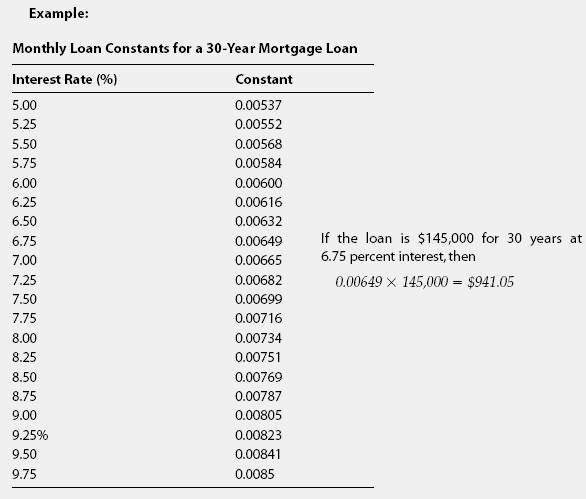# constant

(redirected from Constant term)
Also found in: Dictionary, Thesaurus, Medical, Encyclopedia.

## constantThe annual payment required to pay the principal and interest due on a \$1 loan for a specified repayment term at a specified interest rate.Before the widespread availability of computers and extremely affordable financial calculators,it was common to use mortgage constant tables to calculate monthly payments. One would refer to the table for the proper loan term, such as 30 years, and then within that table find the loan constant for the anticipated interest rate. See the following example. The constant is the monthly payment on \$1. To find out your own monthly payment, you multiply the constant by the number of dollars in your loan, and the answer is what your monthly payments would be.

The Complete Real Estate Encyclopedia by Denise L. Evans, JD & O. William Evans, JD. Copyright © 2007 by The McGraw-Hill Companies, Inc.
References in periodicals archive ?
In the Fixed Effects Model, a different constant term is used for each section while the slope coefficient remains the same.
Basically, the tool chooses the values for the constant term (a) and the slope coefficient (b) so as to minimize ESS.
Equation (37) may be assumed as second-order partial differential equation near the event horizon in general nonstationary black hole since all the coefficients [L.sub.1], [L.sub.2], [L.sub.3], and [L.sub.4] are constant terms when r [right arrow] [r.sub.h]([u.sub.0], [d.sub.0], [[theta].sub.0]), u [right arrow] [u.sub.0], [theta] [right arrow] [[theta].sub.0], and [phi] [right arrow] [[phi].sub.0].
An average inactive participant aged 28 shows a HS score of 1.12 (constant term) indicating that the samples average participant in early adulthood shows rarely any lifestyle impacting health limitations.
The computation overhead Scheme GKD Each group member GKMP  t enc 1 dec AGKTP  1 Gen_[f.sub.t](x) + 1 hash 1 Gen_[f.sub.t](0) + 1 hash SAKDA  2 inv + 1 hash 1 inv + 1 exp +x + 1 hash Our Scheme t Gen_[f.sub.1](x) + 1 hash or 1 Gen_[f.sub.1](0) + 1 hash or 1 Gen_[f.sub.1](x) + 1 hash + 1 enc 1 dec + 1 hash t: the number of group members Gen_[f.sub.d](x): generate a polynomial of degree d  Gen_[f.sub.d](0): restore the constant term of [f.sub.d](x)  inv: modular multiplicative inverse exp: modular exponentiation enc/dec: symmetric encryption/decryption
Evaluation parameters 250 frames 500 frames SSE 3.86 35.27 R-square 0.9989 0.9907 Adjusted R-square 0.9978 0.9815 RMSE 0.1279 0.2656 TABLE 3: Effect of evaluation parameter values of 500-frame thermal image data fitted by model with or without constant term. Evaluation parameters Initial model Improved model (w/o constant term) (w constant term) SSE 35.27 0.89 R-square 0.9907 0.9998 Adjusted R-square 0.9815 0.9995 RMSE 0.2656 0.0422
Clearly, (3) has no constant term because it is a homogeneous exponential equation.
The set of instruments for the GMM estimation includes [z.sub.t], [[??].sup.+.sub.t] and [[??].sup.-.sub.t] with 1 and 2 lags and a constant term. Similar to the above case, the discount factor [delta] is set to 0.999, 0.950, and 0.900 to check the robustness of our estimation results.
[[beta].sub.0] is the constant term while [[epsilon].sub.it] is the error term.
US \$ Period Constant term Regression t=Values R2 = Values (a) coefficient (b) 1 2 3 4 5 Industrial Countries (ICs) 1981-91 148.03 767.86 * 5.72 0.86 * 1991-99 179.19 2298.59 * 17.78 0.90 * 1981-99 167.41 666.18 * 7.06 0.96 * Developing Countries (DCs) 1981-91 39.62 518.13 * 9.70 0.74 * 1991-99 120.92 910.66 * 10.90 0.91 * 1981-99 79.85 302.73 * 4.04 0.90 * World 1981-91 187.64 1286.04 * 7.35 0.86 * 1991-99 305.12 3195.38 * 15.73 0.91 * 1981-99 248.66 960.16 * 6.17 0.95 * India 1981-91 1.01 5.18 * 5.64 0.83 * 1991-99 2.47 15.61 * 10.33 0.92 * 1981-99 1.71 21.07 *** 1.54 0.93 * Y = Export in bill.
This can be explained by the fact that the even leading terms do not have a constant term but only sinusoidal functions which converge faster than the constant term.
Our specific interest is predicates of the form P(x) = [[1 + sign(Q(x))]/2] where Q: [R.sup.k] [right arrow] R is a quadratic polynomial with no constant term, i.e., Q(x) = [[summation].sup.k.sub.i=1] [[alpha].sub.i][x.sub.i] + [[summation].sub.i[not equal to]j] [b.sub.ij][x.sub.i][x.sub.j] for some set of coefficients [a.sub.1],..., [a.sub.n] and [b.sub.11],..., [b.sub.nn].

Site: Follow: Share:
Open / Close